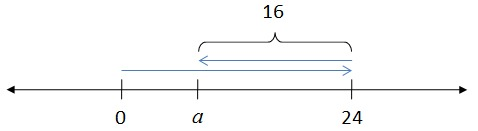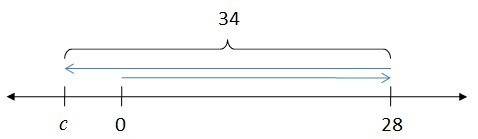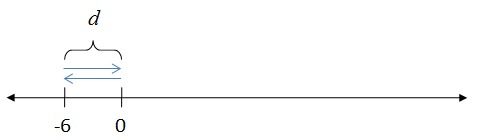# Bookstore Account

Alignments to Content Standards: 7.NS.A.1 7.EE.B.4

1. At the beginning of the month, Evan had \$24 in his account at the school bookstore. Use a variable to represent the unknown quantity in each transaction below and write an equation to represent it. Then represent each transaction on a number line. What is the unknown quantity in each case? 1. First he bought some notebooks and pens that cost \$16.
2. Then he deposited some more money and his account balance was \$28. 3. Then he bought a book for English class that cost \$34.
4. Then he deposited exactly enough money so that he paid off his debt to the bookstore.

2. Explain why it makes sense to use a negative number to represent Evan's account balance when he owes money.

## IM Commentary

Many people like to use debt to help students understand negative numbers. When doing so, it is important to keep in mind that representing debt with negative numbers is a convention, and that it would be possible to talk about debt without ever using negative numbers. For example, if I borrow \$30 from my cousin, I owe her +30 dollars. If I give her \$40, then she owes me +10 dollars. The reason it is so convenient to represent debt with negative numbers is that it allows us to use arithmetic and algebra to keep track of who owes what to whom. Despite the fact that it is convenient to do so, it is not required simply by setting up a problem involving debt.

The purpose of this task is for students to use algebra and the number line to understand why it makes sense that we sometimes represent debt using negative numbers. If we agree that depositing money in an account adds a positive number to the balance, and buying somethings subtracts a positive number from the balance, then it is natural to represent debt with negative numbers.

The number line is an important mathematical model which helps students visualize numbers and make sense of addition and subtraction of numbers. In the middle school grades, it is important for them to develop the ability to perform arithmetic in a more abstract setting, paving the way for the development of algebraic reasoning. Thus in 6th grade they ''Apply and extend previous understandings of arithmetic to algebraic expressions'' (6.EE.A) and in the 7th grade students ''Solve real-life and mathematical problems using numerical and algebraic expressions and equations'' (7.EE.4). This task provides an opportunity for students to use the number line to explain arithmetic with the integers while at the same time representing these transactions with algebraic expressions. In other words, students are both increasing their understanding of arithmetic with numbers while working toward arithmetic with expressions which will be more fully developed in the 8th grade and in high school algebra.

This task assumes that students have already used the number line to represent signed numbers and that they know how to represent addition and subtraction of positive numbers on the number line. In other words, students should know that:

• For every number $a$ on the number line there is a number $-a$ on the other side of zero that is equally distant from 0. These numbers are called opposites or additive inverses.

• To add a positive number $b$ to a number $a$, you move $b$ units to the right of $a$ on the number line.

• To subtract a positive number $b$ from a number $a$, you move $b$ units to the left of $a$ on the number line.

Putting this together with the fact that depositing money in the bookstore account means adding an amount to the account balance, and buying an item from the bookstore means subtracting an amount from the account balance leads us to see why it makes sense to represent debt with negative numbers.

## Solution

• He started with \$24 and spent \$16. Let $a$ represent his account balance after he bought the notebook and pens. Then $$24 – 16 = a$$His new account balance is \$8. • He has \$8 in his account and then he deposited $b$ dollars. His account balance is now \$28. Then $$8 + b = 28$$His deposited \$20 in his account.

• He has \$28 and spent \$34. Let $c$ represent his account balance after he bought the book. Then $$28 – 34 = c$$His new account balance is -\$6. • He started with an account balance of -\$6 and paid the debt off so his account balance is 0. If $d$ is the amount money he deposited to pay off his debt, then $$-6 + d = 0$$He deposited \\$6.

1. It makes sense to use a negative number to represent Evan's account balance when he owes money. Here are two ways to look at it:

(1) We always take the current account balance and add a positive number to that if he makes a deposit or subtract a positive number from that if he buys items at the bookstore. If he spends more than he has in his account, then we are subtracting a bigger number from a smaller number, and the result is negative.

(2) We can also see in this context that if he deposits just enough money to pay off his debt, that means we are adding a positive number to his account balance and the result is 0. If you add two numbers together to get 0, then the numbers must be opposites. The opposite of a positive number is a negative number, so it makes sense to represent his account balance with a negative number when he owes money to the bookstore.